# Electronics and Communication Engineering - Exam Questions Papers

1.

The circuit given below is: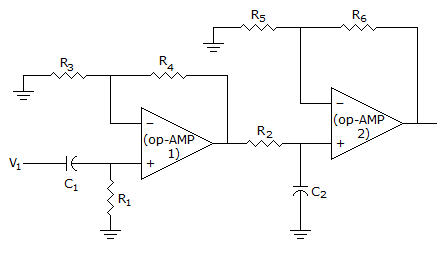A. Band stop filter B. Band pass filter C. Oscillator circuit D. All pass filter

Explanation:

The given circuit is Band pass filter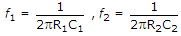.

2.

Rank of the matrix: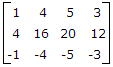A. 3 B. 2 C. 1 D. 4

Explanation:

The given matrix is equivalent to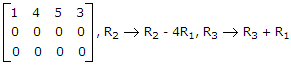,

Thus rank is 1.

3.

His rather casual remarks on politics __________ his lack of seriousness about the subject.

 A. masked B. belied C. cherish D. conserve

Explanation:

The key words in the statement are 'casual remarks' and 'lack of seriousness'.

The blank should be filled with a word meaning 'showed' or 'revealed'. Hence, 'betrayed' is the correct answer.

4.

The resolution of a 4 bit counting ADC is 0.5 volts. For an analog input of 6.6 volts, the digital output of the ADC will be

 A. 1011 B. 1101 C. 1100 D. 1110

Explanation:

Resolution =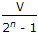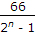= 0.5

2n = 14 = (1110)

n = 4 bits.

5.

For the power amplifier circuit shown below, the maximum power dissipated by both output transistor is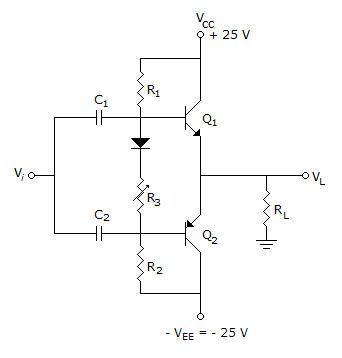A. 9.66 W B. 30.11 W C. 31.66 W D. 33.66 W

The max. power dissipated by both output transistor is max. P2 =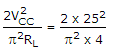= 31.66 W.